# Exploratory-analysis-II

Data visualization, part 2. Code for Quiz 8.

1. Load the R package we will use.
``````library(tidyverse)
library(patchwork)
``````
1. Quiz Questions
• Replace all the ???s. These are answers on your moodle quiz.

• Run all the individual code chunks to make sure the answers in this file correspond with your quiz questions.

• After you checks all of your code chunks run then you can knit it. It won’t knit until the ??? are replaced

• The quiz assumes that you have watched the videos, downloaded (to your examples folder) and worked through the exercises in exercises_slides-50-61.Rmd

3.Pick one of your plots to save as your preview plot. Use the ggsave command at the end of the chunk of the plot that you want to preview.

# Question: modify slide 51

• Create a plot with the mpg dataset

• assign the variable displ to the x-axis

• assign the variable hwy to the y-axis

• add facet_wrap to split the data into panels based on the manufacturer

``````ggplot(mpg) +
geom_point(aes(x = displ, y = hwy)) +
facet_wrap(vars(manufacturer))
``````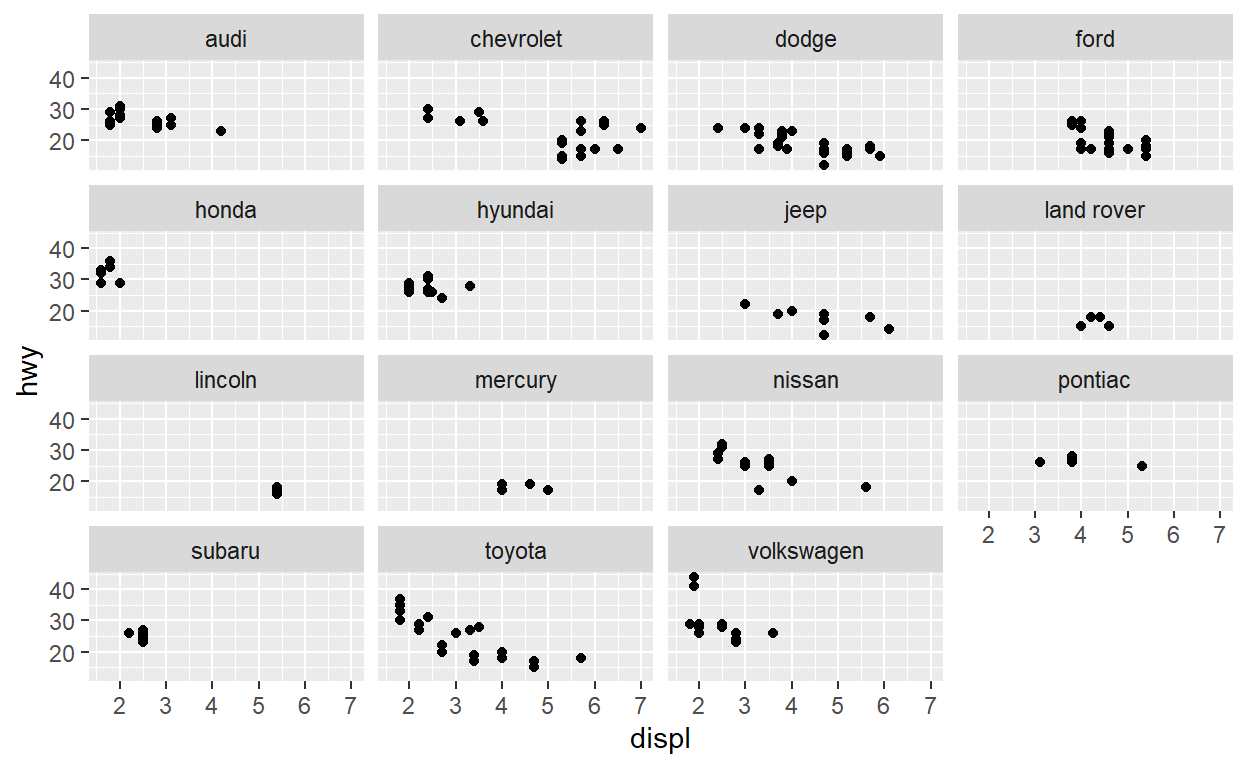# Question: modify facet-ex-2

• Create a plot with the mpg dataset

• add bars with with geom_bar

• assign the variable manufacturer to the y-axis

• add facet_grid to split the data into panels based on the class

• let scales vary across columns

• let space taken up by panels vary by columns

``````ggplot(mpg) +
geom_bar(aes(y = manufacturer)) +
facet_grid(rows = vars(class), scales = "free_y", space = "free_y" )
``````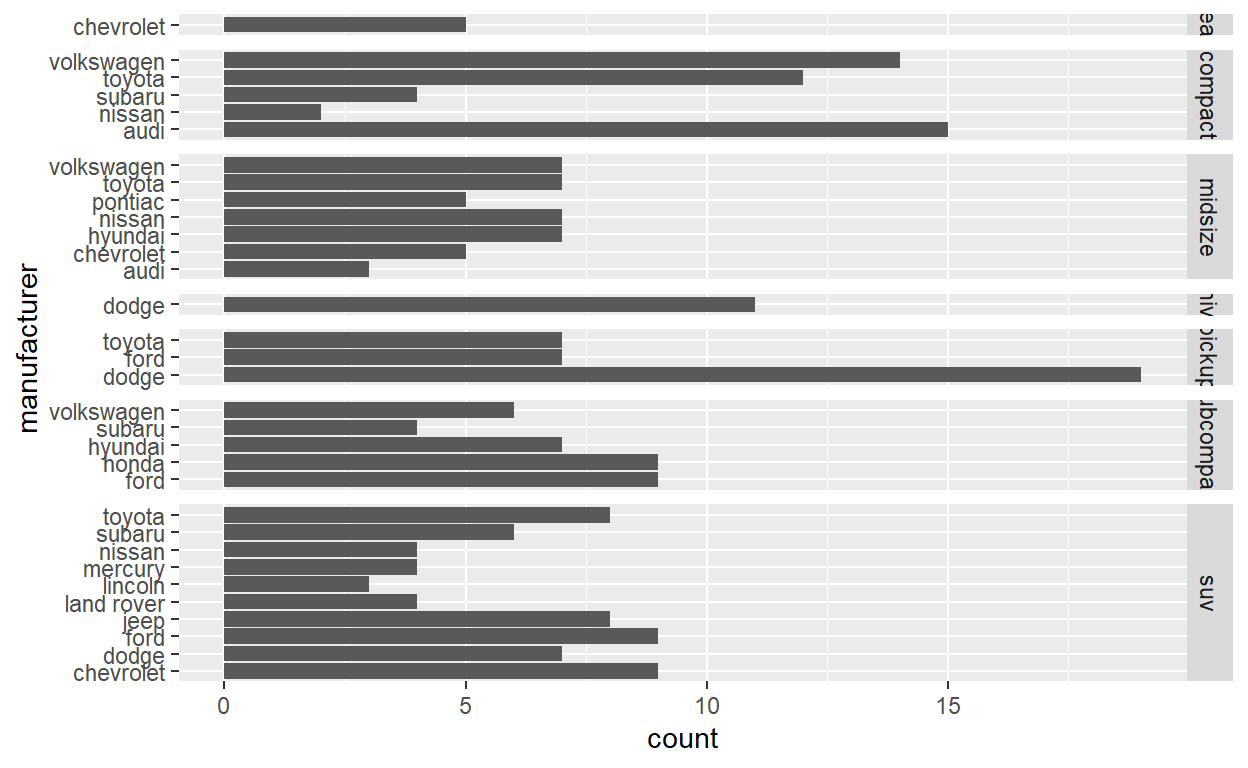# Question: spend_time

``````spend_time <- read_csv("spend_time.csv")
``````

• extract observations for 2011

• THEN create a plot with that data

• ADD a barchart with with geom_col

• assign activity to the x-axis

• assign avg_hours to the y-axis

• assign activity to fill

• ADD scale_y_continuous with breaks every hour from 0 to 6 hours

• set subtitle to Avg hours per day: 2011

• set x and y to NULL so they won’t be labeled

• assign the output to p1

• display p1

``````p1  <- spend_time %>% filter(year == "2011")  %>%
ggplot() +
geom_col(aes(x = activity, y = avg_hours, fill = activity)) +
scale_y_continuous(breaks = seq(0, 6, by = 1)) +
labs(subtitle = "Avg hours per day: 2011", x = NULL, y = NULL)

p1
``````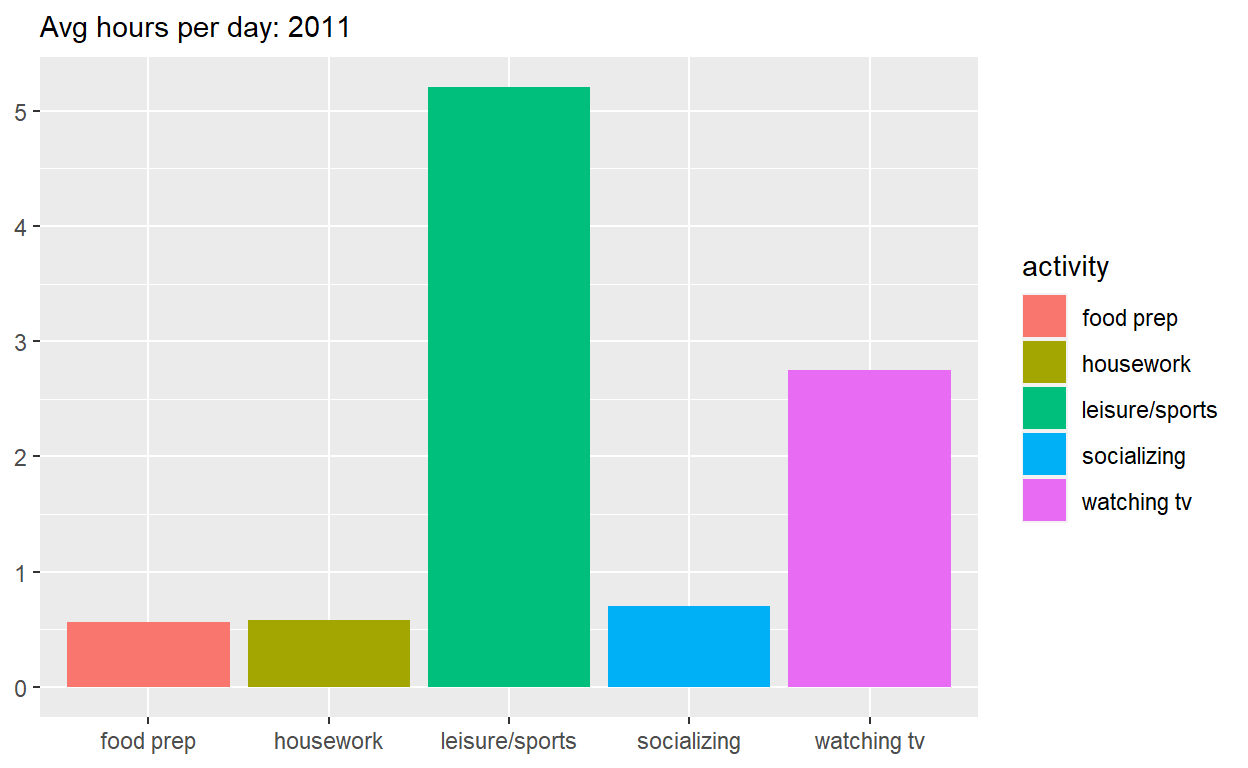• THEN create a plot with it

• ADD a barchart with with geom_col

• assign year to the x-axis

• assign avg_hours to the y-axis

• assign activity to fill

• set subtitle to “Avg hours per day: 2010-2019”

• set x and y to NULL so they won’t be labeled

• assign the output to p2

• display p2

``````p2  <- spend_time  %>%
ggplot() +
geom_col(aes(x = year, y = avg_hours, fill = activity)) +
labs(subtitle  = "Avg hours per day: 2010-2019", x = NULL, y = NULL)

p2
``````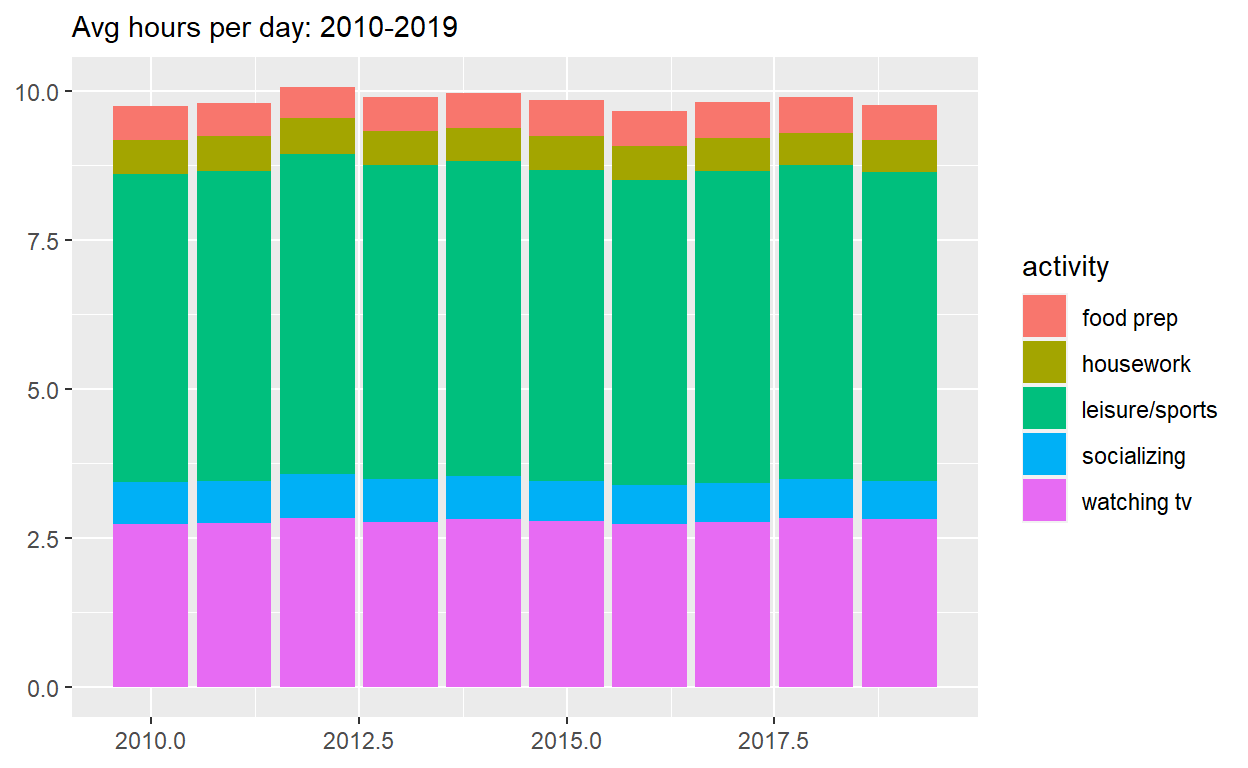Use patchwork to display p1 on top of p2

-assign the output to p_all

• display p_all
``````p_all  <-  p1 / p2

p_all
``````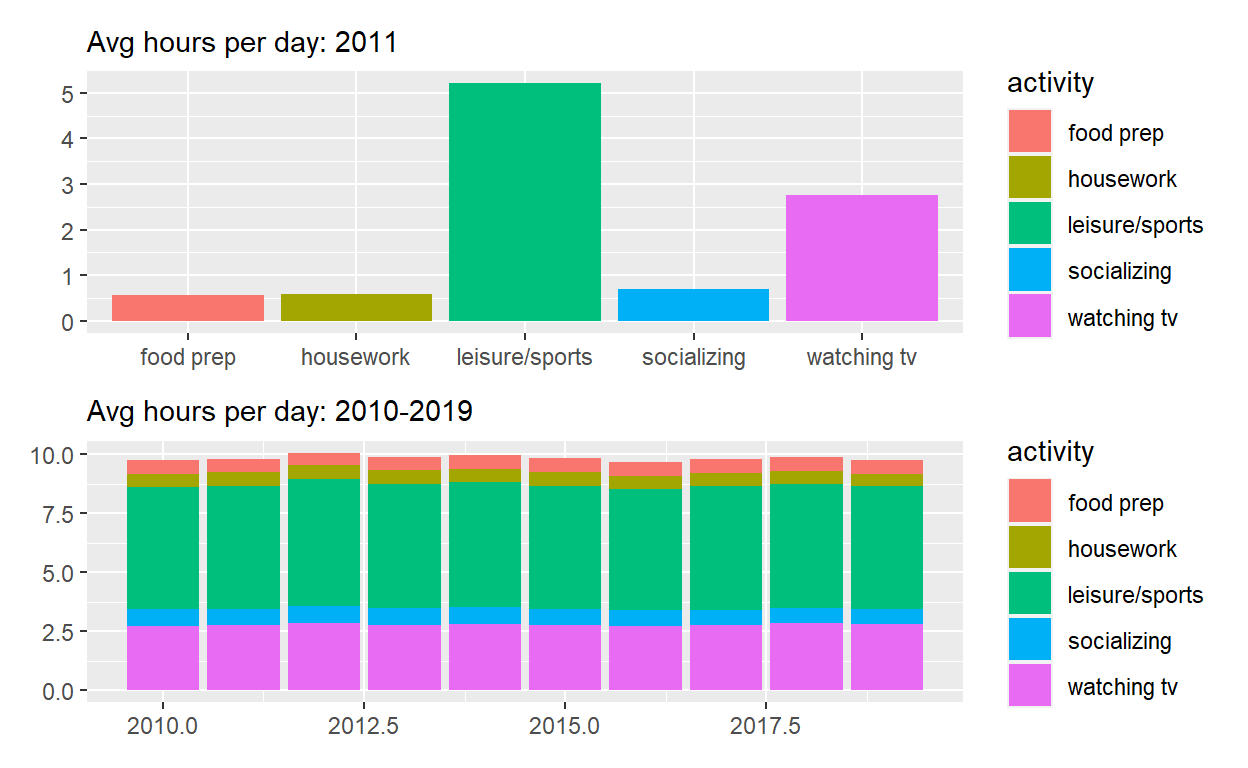• AND set legend.position to ‘none’ to get rid of the legend

• assign the output to p_all_no_legend

• display p_all_no_legend

``````p_all_no_legend  <- p_all & theme(legend.position = 'none')
p_all_no_legend
``````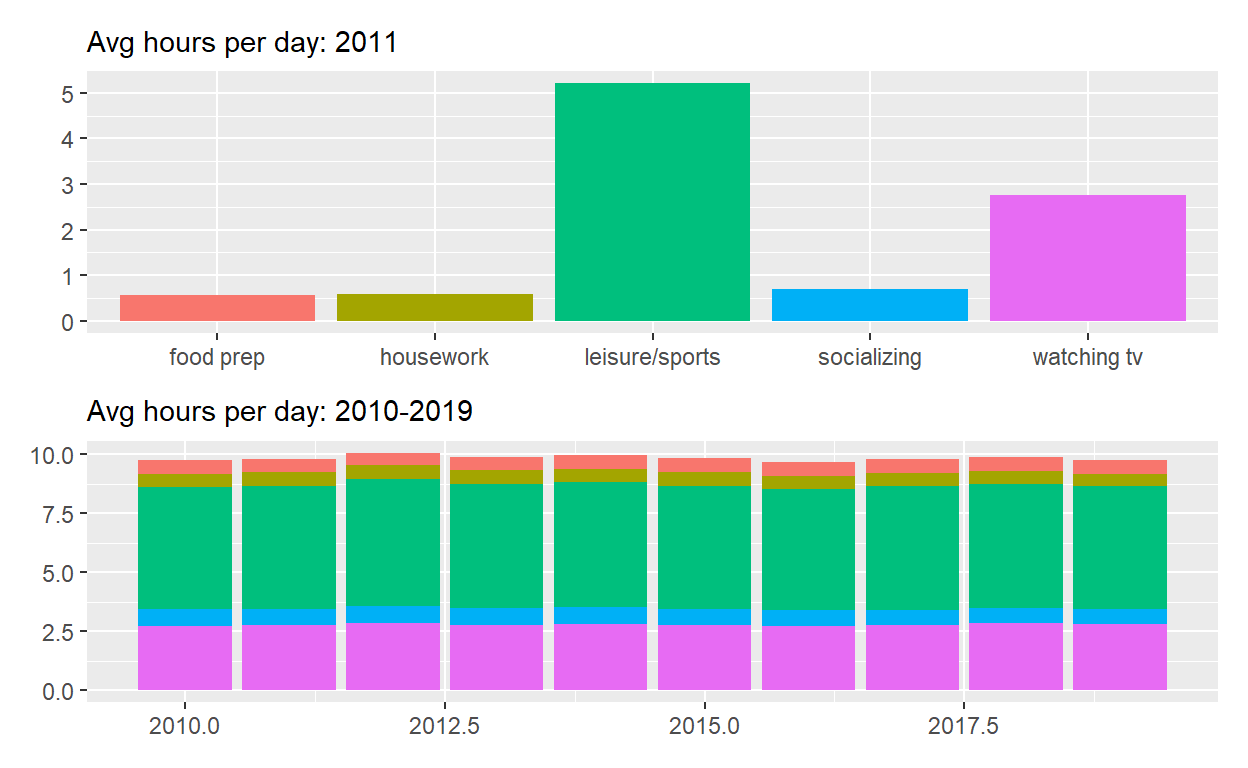-caption to “Source: American Time of Use Survey, https://data.bls.gov/cgi-bin/surveymost?tu

``````p_all_no_legend  +
plot_annotation(title = "How much time Americans spent on selected activities",
caption = "Source: American Time of Use Survey, https://data.bls.gov/cgi-bin/surveymost?tu")
``````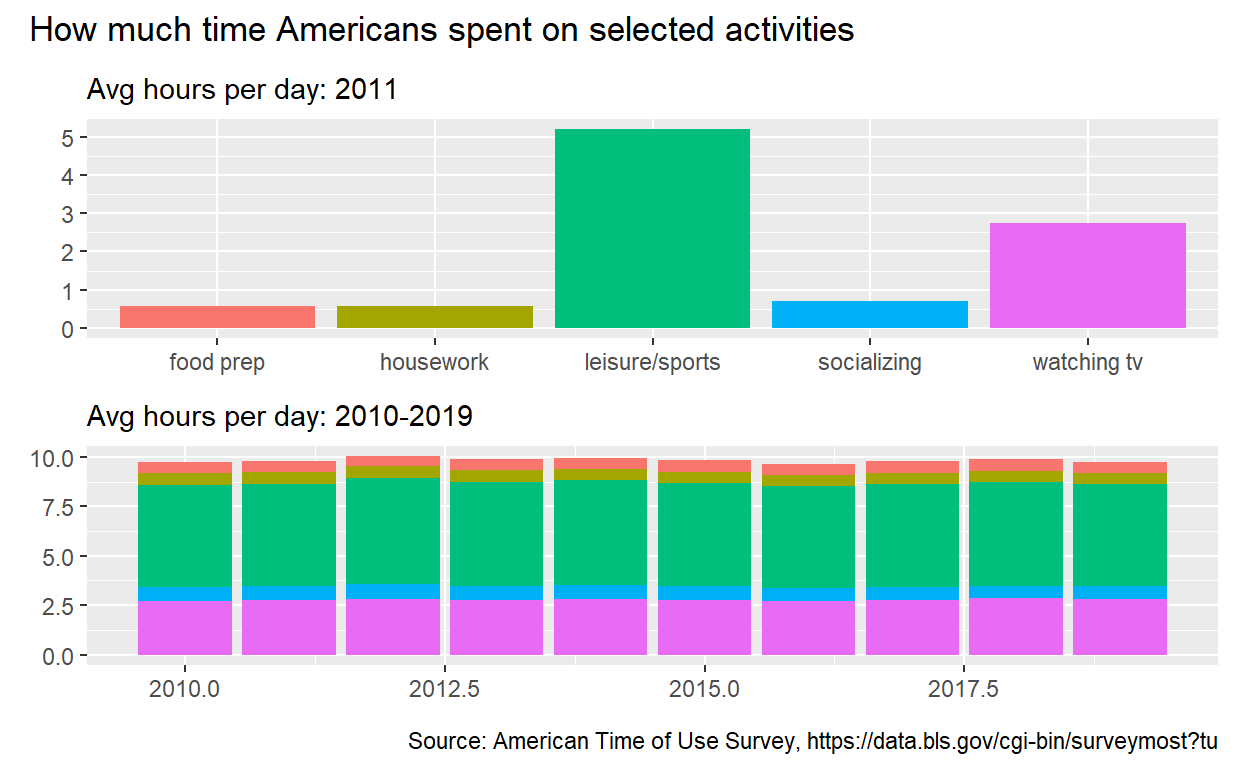# Question: Patchwork 2

use spend_time from last question patchwork slides

• extract observations for leisure/sports

• THEN create a plot with that data

• assign year to the x-axis

• assign avg_hours to the y-axis

• assign year to the x-axis

• assign avg_hours to the y-axis

• ADD breaks on for every year on x axis with with scale_x_continuous

• set subtitle to Avg hours per day: leisure/sports

• set x and y to NULL so x and y axes won’t be labeled

• assign the output to p4

• display p4

``````p4  <-
spend_time %>% filter(activity == "leisure/sports")  %>%
ggplot() +
geom_point(aes(x = year, y = avg_hours)) +
geom_smooth(aes(x = year, y = avg_hours)) +
scale_x_continuous(breaks = seq(2010, 2019, by = 1)) +
labs(subtitle = "Avg hours per day: leisure/sports", x = NULL, y = NULL)
p4
``````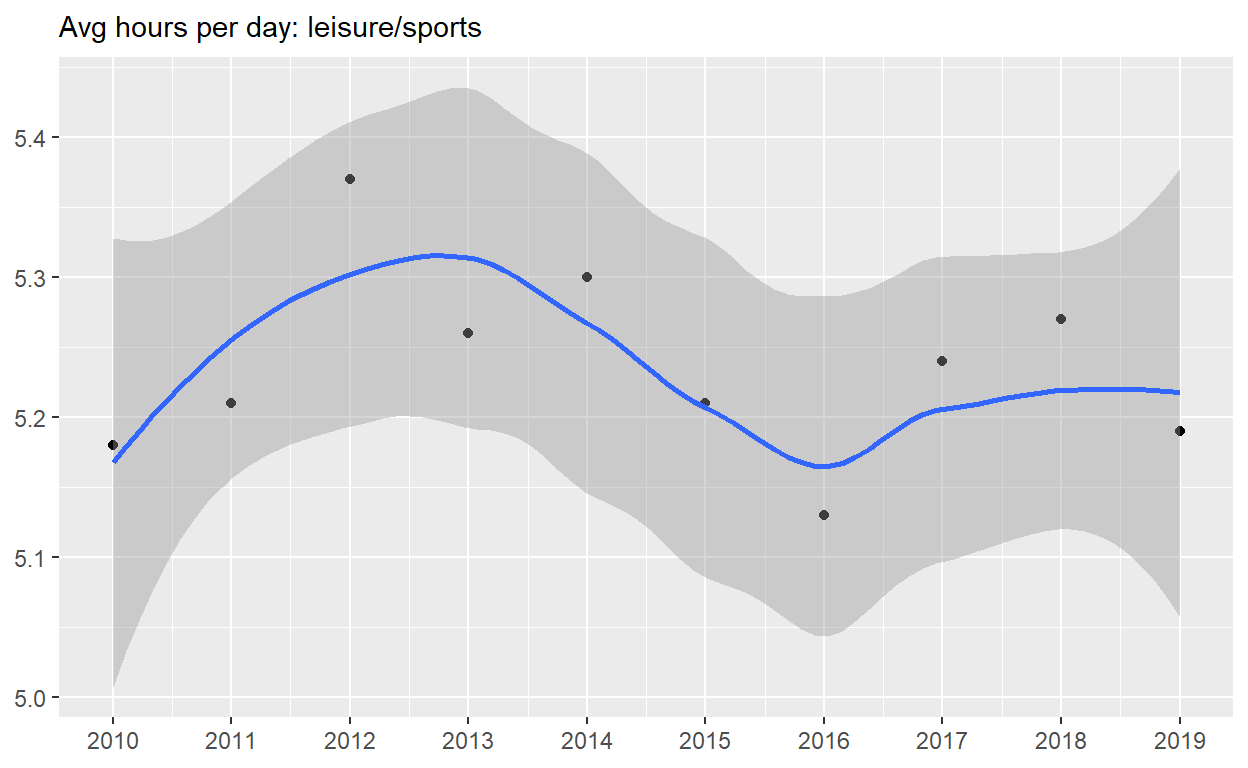• ADD coord_cartesian to change range on y axis to 0 to 6

• assign the output to p5

• display p5

``````p5 <-  p4 + coord_cartesian(ylim = c(0, 6))
p5
``````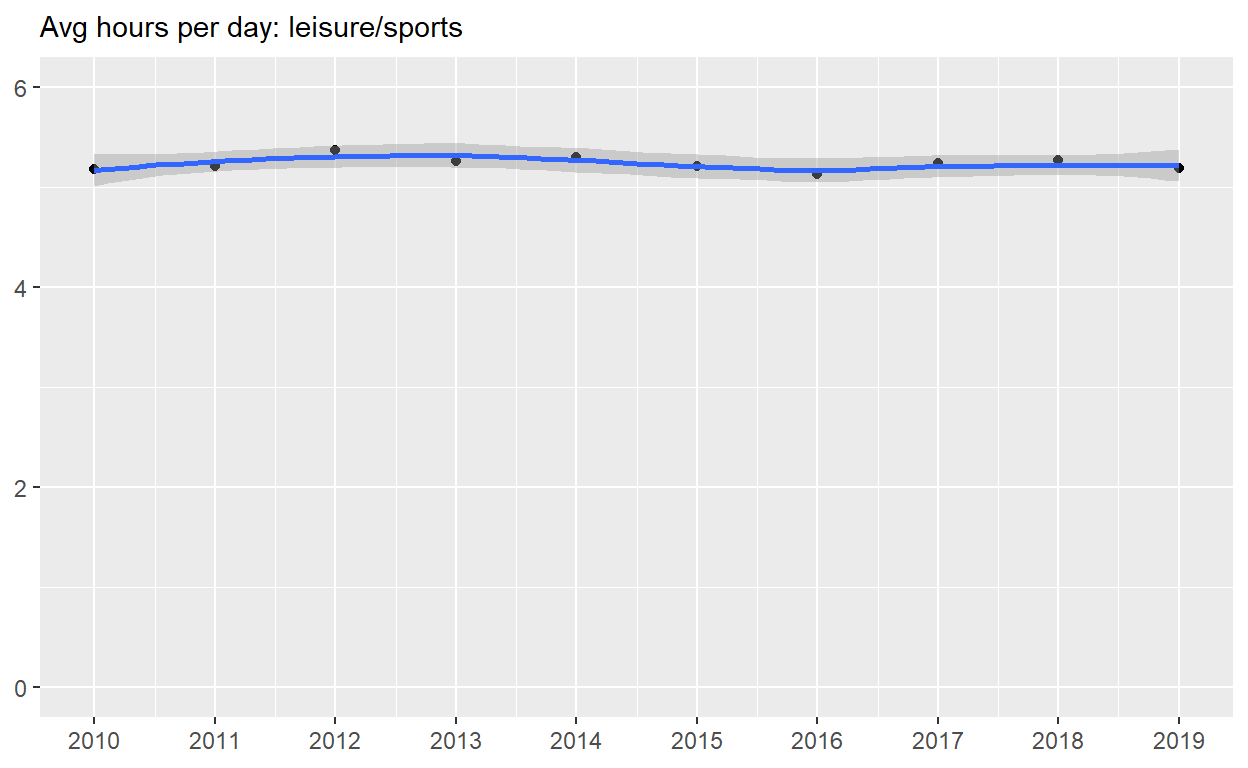• create a plot with that data

• assign year to the x-axis

• assign avg_hours to the y-axis

• assign activity to color

• assign activity to group

• assign year to the x-axis

• assign avg_hours to the y-axis

• assign activity to color

• assign activity to group

• ADD breaks on for every year on x axis with with scale_x_continuous

• ADD coord_cartesian to change range on y axis to 0 to 6

• set x and y to NULL so they won’t be labeled

• assign the output to p6

• display p6

``````p6   <-
spend_time  %>%
ggplot() +
geom_point(aes(x = year, y = avg_hours, color = activity, group = activity)) +
geom_smooth(aes(x = year, y = avg_hours, color = activity, group = activity)) +
scale_x_continuous(breaks = seq(2010, 2019, by = 1)) +
coord_cartesian(ylim = c(0, 6)) +
labs(x = NULL, y = NULL)

p6
``````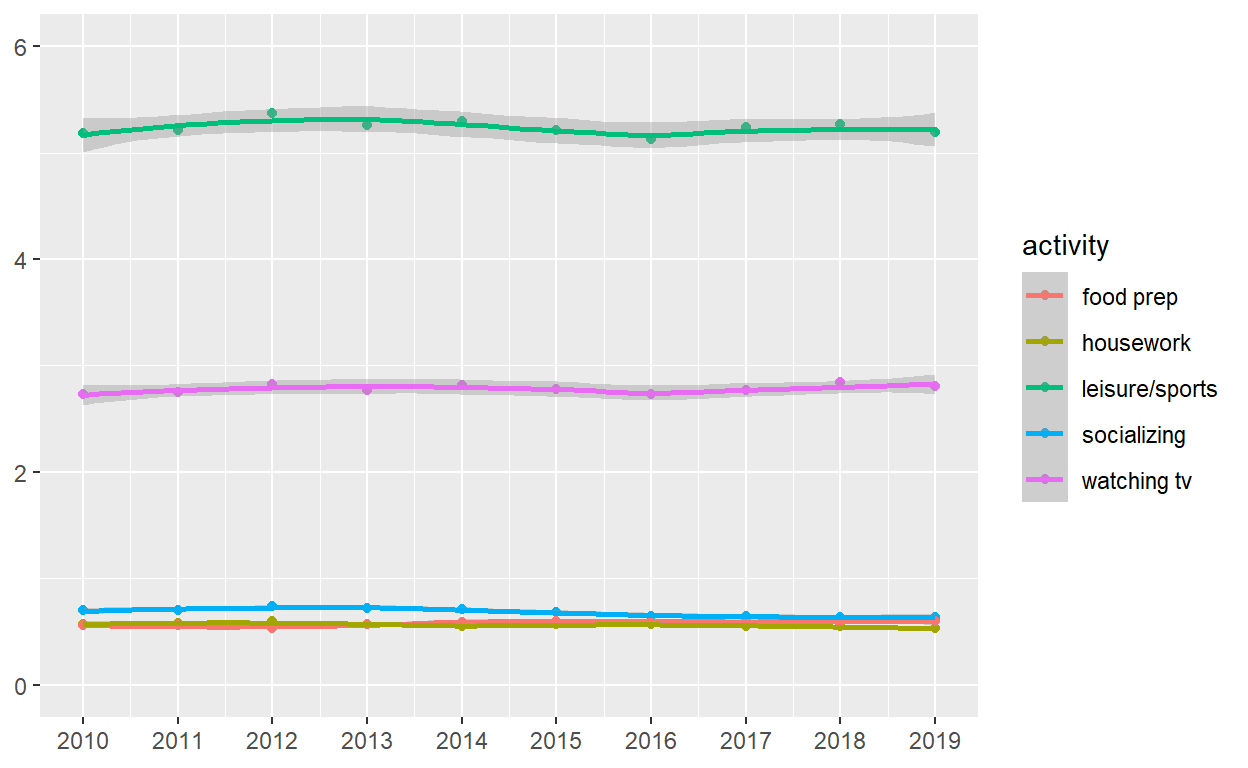Use patchwork to display p4 and p5 on top of p6

``````( p4 | p5 ) / p6
``````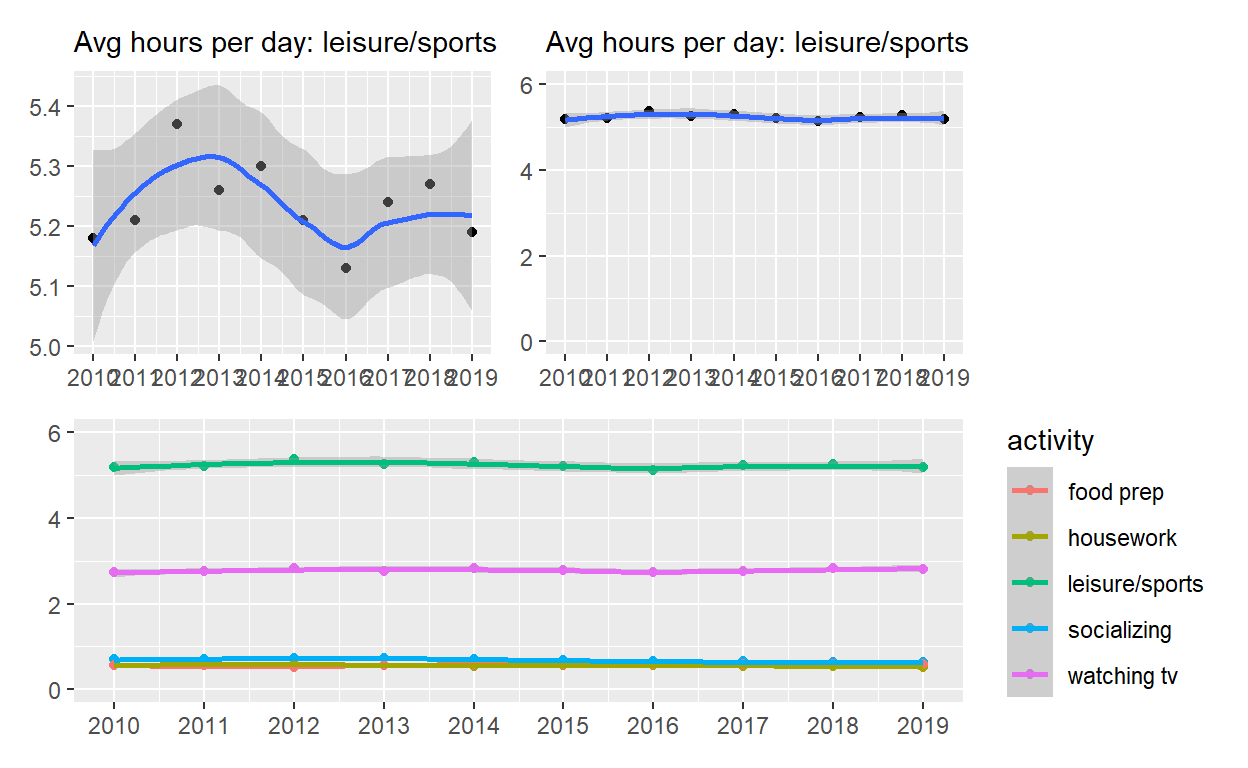``````ggsave(filename = "preview.png",
path = here::here("_posts", "2021-04-06-exploratory-analysis-ii"))
``````